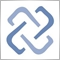# Discussion of article "Practical application of neural networks in trading. Python (Part I)"Moderator
228710

New article Practical application of neural networks in trading. Python (Part I) has been published:

In this article, we will analyze the step-by-step implementation of a trading system based on the programming of deep neural networks in Python. This will be performed using the TensorFlow machine learning library developed by Google. We will also use the Keras library for describing neural networks.

Let us consider some points related to data preparation for neural network training.

• For decision making, we will use two neural networks for opening positions in one direction
• According to the previous point, the training data should be divided into two groups - one for each direction.
• As in the previous system, the first neural network will be trained to build indicators similar to standard technical indicators. We used this solution in the previous system because we used self-written indicators and we didn't want to overload the working Expert Advisor. Python is used because only quotes can be received from the terminal. To prepare data for the neural network, we need to build these indicators in a Python script. By teaching the neural network to build such indicators, we eliminate the need to duplicate them in the script.
• The second neural network builds the signal indicator, based on which we create a trading strategy.
• The neural network will be trained for the EURUSD H1 chart.
• As a result, to build the system, we will need to prepare two neural networks for buying and two networks for selling. Thus, four neural networks will work in the system.

Author: Andrey Dibrov